# The Computer in Sound System Design (Aug. 1970)

 Home | Audio Magazine | Stereo Review magazine | Good Sound | Troubleshooting Departments | Features | ADs | Equipment | Music/Recordings | History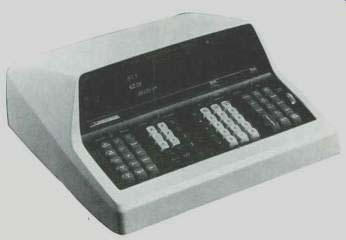by DON DAVIS [Director of Commercial Sound Products, Altec Lansing Division of LTV Ling Altec. ] As we enter the decade of the Seventies more and more professional sound contractors are becoming capable of truly engineering a sound reinforcement system. They think of rooms in terms of shape, average absorption coefficients, critical distance; and of loudspeakers in terms of efficiency, directivity, and uniformity of dispersion. It is refreshing to hear engineering discussions rather than salesman's platitudes regarding equipment performance. This article stresses universal performance parameters that must be met. AN ACCURATE KNOWLEDGE Of a room's size and shape, its reverberation characteristics, and its ambient noise level allows the modern sound contractor to evaluate quickly the advantages and disadvantages of using one of the three basic approaches to sound system design, namely: 1. High-level single-source system. 2. High-level overhead distributed system. 3. Combinational system. The sound contractor first measures or calculates the following parameters: 1. Reverberation decay time. 2. Average absorption coefficient. 3. Ambient noise level. 4. Room constant. 5. Critical distance. 6. Distance between the performer and the most remotely located listener. 7. Equivalent acoustic distance. 8. Needed acoustic gain 9. Potential acoustic gain. 10. Electrical power required. 11. Time delay. 12. Distribution requirements. He is then fully equipped to predict accurately and precisely what can be done with the sound system ( and even more important, what should not be done ) and is capable of delivering what he predicts. How Sound Behaves Outdoors In outdoor conditions sound essentially follows the inverse-square-law attenuation rate: 20 log10 = Dn/Df =-dB loss (1) Where Dn=the starting point Df=the distant point By arranging the distances so as to produce a fractional number of less than 1, the negative sign is automatically inserted when used in a computer. Figure 1 illustrates the inverse-square-law attenuation rate of sound with increasing distances. How Sound Behaves in a Room As a listener moves away from a loudspeaker in a room he becomes aware of several phenomena: First, the sound initially drops in loudness with increasing distance and then reaches a relatively steady loudness. When near the . loudspeaker it is easy to localize it, but at a distance, location of the loudspeaker can be difficult if the listener closes his eyes. Finally, at a distance from the loudspeaker, high frequencies drop off rather rapidly. For any given listener position, then, this means that we require a reasonably accurate calculation of: 1. The attenuation of sound between the loudspeaker and the listener. 2. The ratio of direct sound to reflected ( reverberant sound) at the listener's ears. 3. The expected change in signal balance due to humidity, air absorption, etc., at the listener's position compared to some closer reference distance. When the loudspeaker or other sound source is moved indoors, several other parameters must be taken into consideration simultaneously. The directivity of the loudspeaker will, in a large part, determine how much sound is concentrated on the audience and how much strikes other surface areas first. How much each of the surface areas reflects becomes of importance as well as the distances involved in the reflections. So in addition to our knowledge of inverse-square-law attenuation we need to add: 1. The loudspeaker's directivity factor known as Q. 2. The room's acoustical characteristics known as the Room Constant (R) . Loudspeaker Directivity Loudspeaker directivity is defined either as the directivity index (D,) or the directivity factor (Q) . To visualize the basic meaning of these parameters let's examine three basic directivity situations: 1. A point source with a perfect spherical radiation pattern. 2. A point source with a perfect hemispherical radiation pattern. 3. A point source with a radiation pattern that is 90° in the vertical axis and 90° in the horizontal axis. We will, in all three cases, assume that the point source is 100 per cent efficient (e.g., 1 electrical watt produces 1 acoustic watt) and that all SPL measurements are to be taken at 4 feet from the source). In the first instance, we can visualize a perfect sphere and as we intrude a small measuring microphone into its four-foot radius from any angle we would expect to read: [Michael Rettinger: Practical Electro-Acoustics, Chemical Publishing Co. Inc., New York, 1955. ]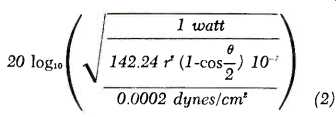eq (2) =dB SPL for 100% efficient source at 4 ft. where 142.24 = a constant derived from a density of air times the velocity of sound in air times 2 pi (English units )--assuming T = 20°C, atmos. Pressure = 760 mm of Hg) r = distance from sound source e = angle subtended by the spherical surface area. Therefore:eq (3) If we apply the same formula to the hemispherical sound source we find that at 4 feet anywhere on the surface of the hemisphere, we can measure:eq (4) We are now in a position to define the directivity index (D,). A quick inspection reveals that simply by confining the radiated power (1 acoustic watt) to a smaller radiated angle (from a spherical radiation pattern to a hemispherical radiation pattern) we benefit by a 3.01-dB increase in our SPL. This increase is the Dr. Therefore, a sound source with a perfect spherical radiation pattern would exhibit a D1 of 0 dB, and a sound source with a perfect hemispherical radiation pattern would exhibit a D1 of 3.01 dB. Once again, let's use the formula to examine the 90-deg. subtended angle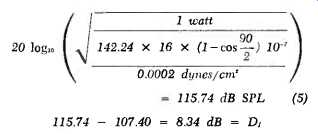eq (5) The directivity factor (Q) is found by the formula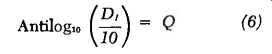eq (6) As can be seen, Q is simply the power ratio of the directivity index. We can now list these parameters in a table, plus other angles of interest. See Fig. 2. It is of interest to sound system engineers that the devices he deals with have the following range of D, and Q. See Fig. 3. [Sound Systems Design, Section B, Manual of Sound Systems, Western Electric Co., 1949.]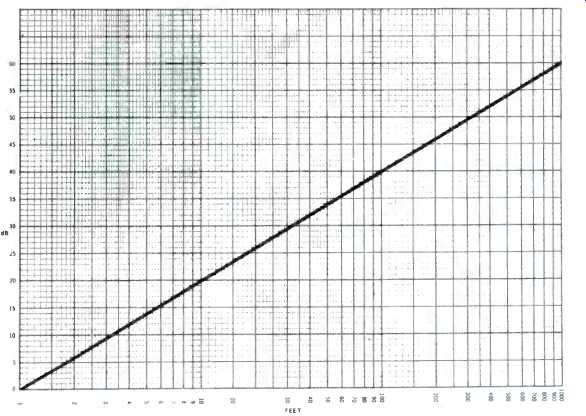Fig. 1--Inverse-square-law attenuation from 1 to 1000 ft.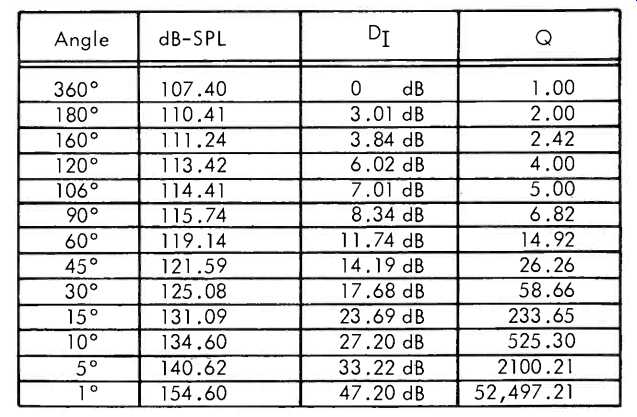Fig 2--SPL in dB at 4 feet from a speaker of 100% efficiency at 1-watt level, radia ing into various angles. The Room Constant When the internal volume (V) of an enclosed space, the total surface area of the boundary surfaces (S), and the reverberation decay time for 60-dB RT is known, it is a simple calculation to obtain the average absorption coefficient (a) of the total room:a can also be calculated, as it must be in the case of the building still on the drawing board, by considering each individual surface area (s) that consists of a different surface material. By means of well established list of absorption coefficients (a), each surface area may be multiplied by its absorption coefficient. The individual surface areas times their individual absorption coefficients can then be summed. With the addition of other objects in the space in terms of sa, the complete total can be divided by the total boundary surface area to obtain a. One such table is shown in Fig. 4.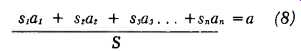eq (8) At this stage, if it is a building on the drawing board, the sound contractor calculates the RT for each of the frequencies for which he has an accurate a by using:eq (9) He also calculates the room constant: eq(10) It can be seen that R is a handy one number value indicating the "liveness" or "deadness" of an acoustic environment.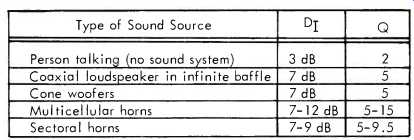Fig. 3--D1 and Q rating for typical sound sources.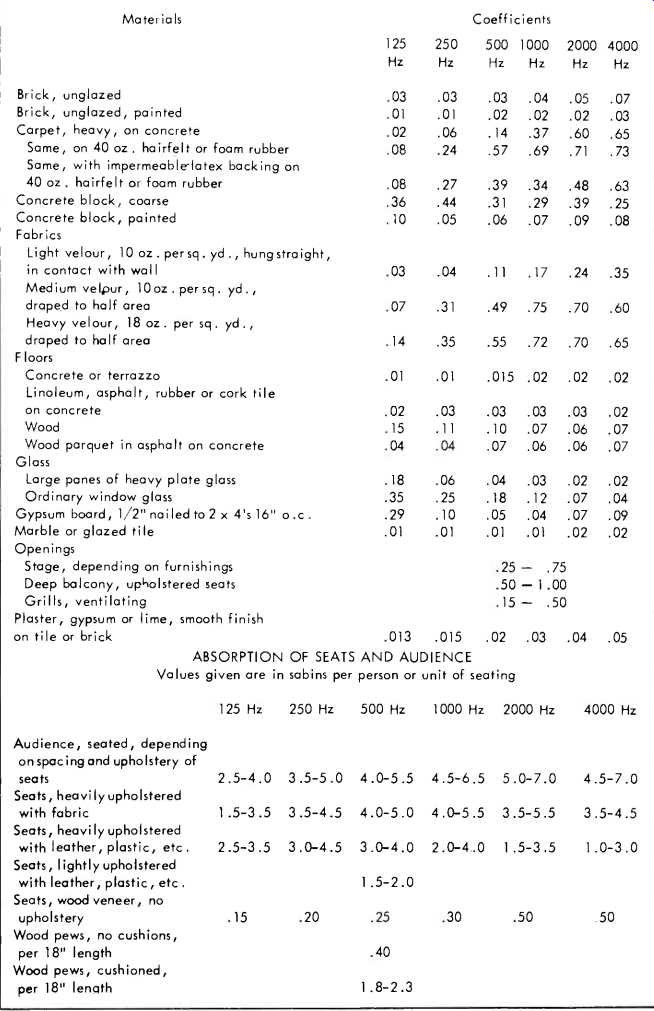-------- SOUND ABSORPTION COEFFICIENTS OF GENERAL BUILDING MATERIALS AND FURNISHINGS Complete tables of coefficients of the various materials that normally constitute the interior finish of rooms may be found in the various books on architectural acoustics. The following short list will be useful in making simple calculations of the reverberation in rooms. Attenuation of Sound with Increasing Distance in an Enclosed Space Using the information developed above, the formula for the attenuation of sound with increasing distance in an enclosed space is not difficult to comprehend or utilize.Eq (11) where Q = the directivity factor r = the distance from the source R = the room constant For the purpose of illustration, let's examine an auditorium where the V = 500,000 ft^3 S = 100,000 ft^2 a=0.12 And the loudspeaker's directivity factor Q = 5.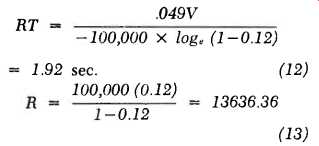Eq (12) Eq (13) We can therefore plot for each increase in r of one foot, the difference between the readings as shown in Fig. 5.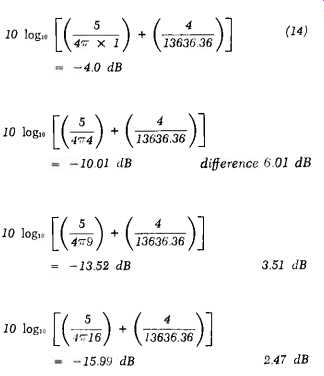Eq. (14) Finding the Ratio of Direct to Reverberant Sound It can be seen clearly that initially every time the distance is doubled, the difference is approximately 6 dB until the distance begins to approach 34 feet. Beyond 34 feet, even if we go to 340 feet we will not encounter any more significant attenuation. Why does sound behave in this manner? What happens at 34 feet? What happens is that the reflected sound at 34 feet is equal in energy to the direct sound. In acoustical terms the distance from the loudspeaker to 34 feet is called the "free field" and the distance beyond 34 feet is called the "reverberant field." See Fig. 6. The distance at which this occurs and at which the direct sound energy from the loudspeaker is equal to the reflected sound energy is called the "critical distance" (D.). Fortunately, there is a much easier way to get D, than by incrementing the formula above:Eq. (15) where Q = S a = the familiar directivity factor. the total boundary surface area. the average absorption coefficient. If D_c by definition is the distance at which the direct sound energy equals the reflected sound energy, and if the direct sound energy (by definition, not undergoing reflection) continues to follow the inverse-square law while the reverberant sound energy tends to remain at a constant level relative to the direct sound level at DT, then we can state that at 2 D_c the ratio of direct-to-reverberant sound is-6 dB and at 4 D_c the ratio of direct-to-reverberant sound is -12 dB. While the human ears coupled to that marvelous real-time analyzer, the human brain, can and does differentiate between the direct and reverberant sound long after the ratio becomes less than 1 to 1, even they can not accurately do so after the ratio exceeds -12 dB. In literally hundreds of high-quality sound reinforcement installations it has been proven that 4D, is the maximum distance any loudspeaker should attempt to project sound if the listener is expected to understand it. [Hewlett Packard, Acoustics Handbook, Application Note 100, Nov. 1968.]Fig. 5--Increments of R in feet.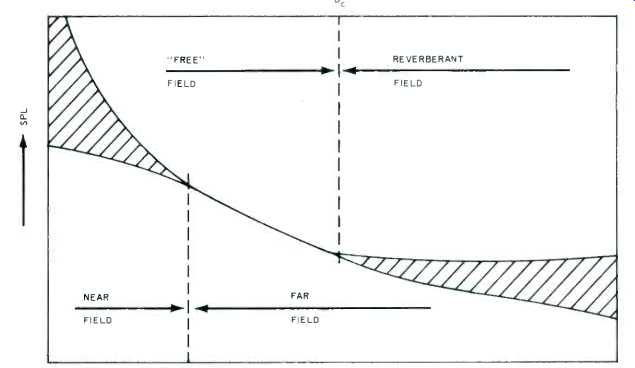Fig. 6--Definition of acoustic fields.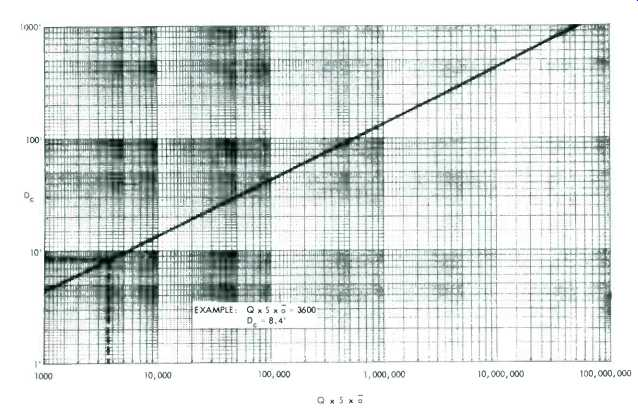Fig. 7--Nomograph for finding critical distance (Dc) It is also interesting to consider that if the sound system microphone and the sound system loudspeaker are separated by Dc, then the microphone can be carried anywhere in the reverberant field without danger of encountering an increased level that will cause acoustic feedback. Further, it can be seen that in a given room where S remains fixed the only way to change Dc is to change either a or Q. One of the easiest ways to change the Q of a loudspeaker is to stack two of them in a vertical line. If cones are used, the Q will shift continuously with frequency. If horns are used, it is possible to find wide frequency areas where Q is relatively under control. One of the real indications of a professional sound contractor is his knowledge and use of the loudspeaker's Q in designing complex loudspeaker arrays. To change a requires increasing the total number of sabins (sa) in the space. The Dr formula allows the sound engineer to examine this change in detail for each space he works in. Figure 7 allows the quick calculations of Dc from Q, S, and a. ============= (Audio magazine, Aug. 1970) Also see: Computer-Aided Audio Calculations (Nov. 1983) Computer-Aided Filter Design (Nov. 1983) Time Delay Spectrometry (TDS) Computing (Nov. 1983)

Updated: Wednesday, 2019-03-27 9:09 PST